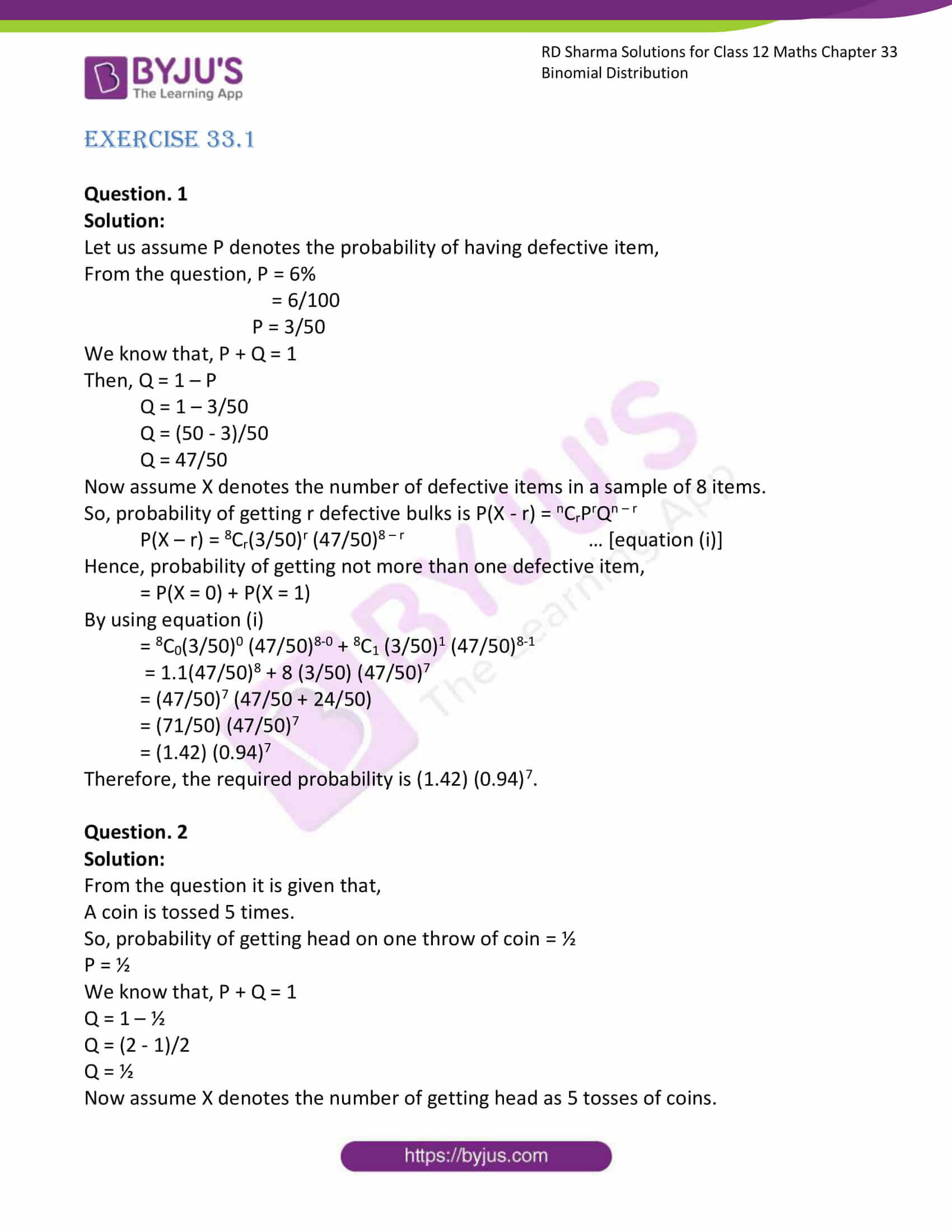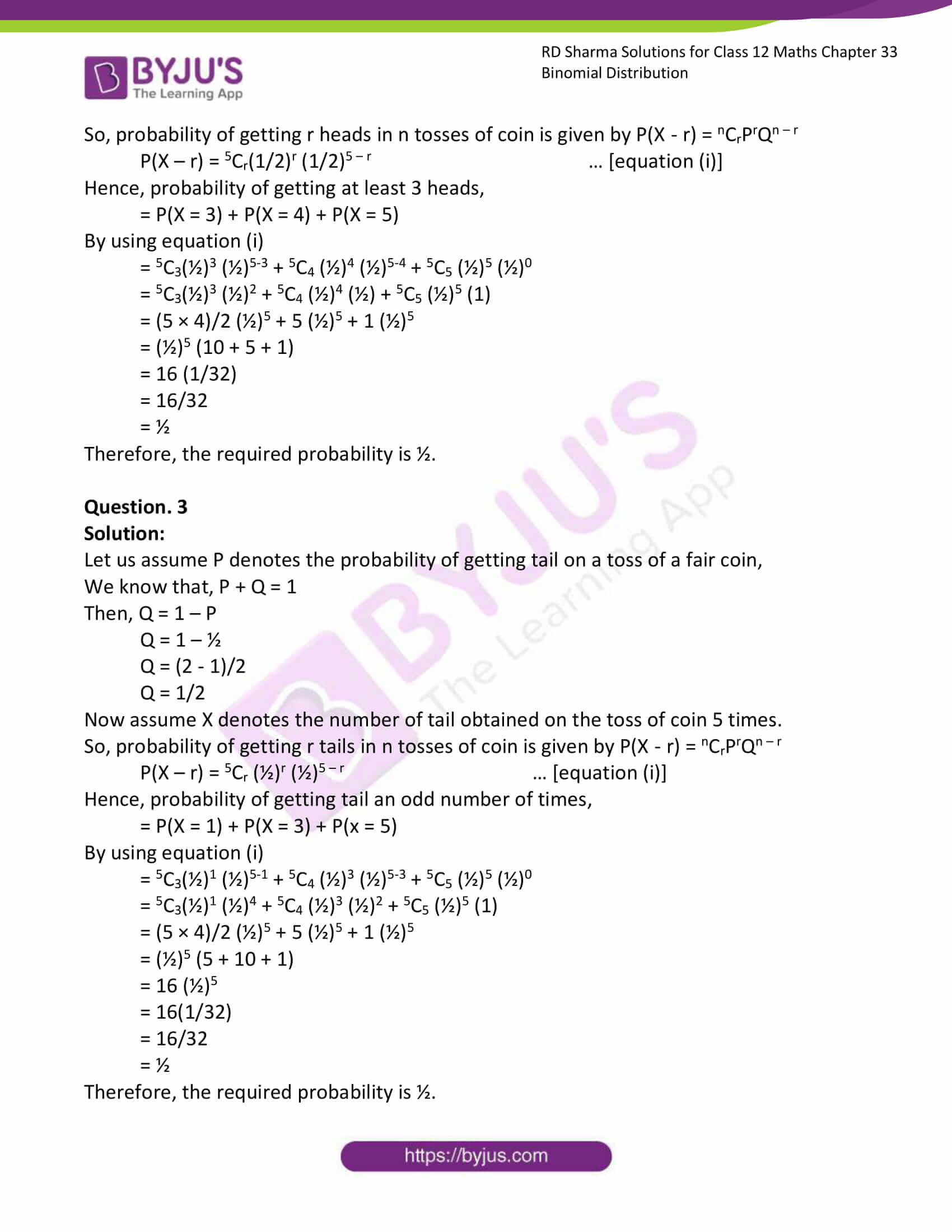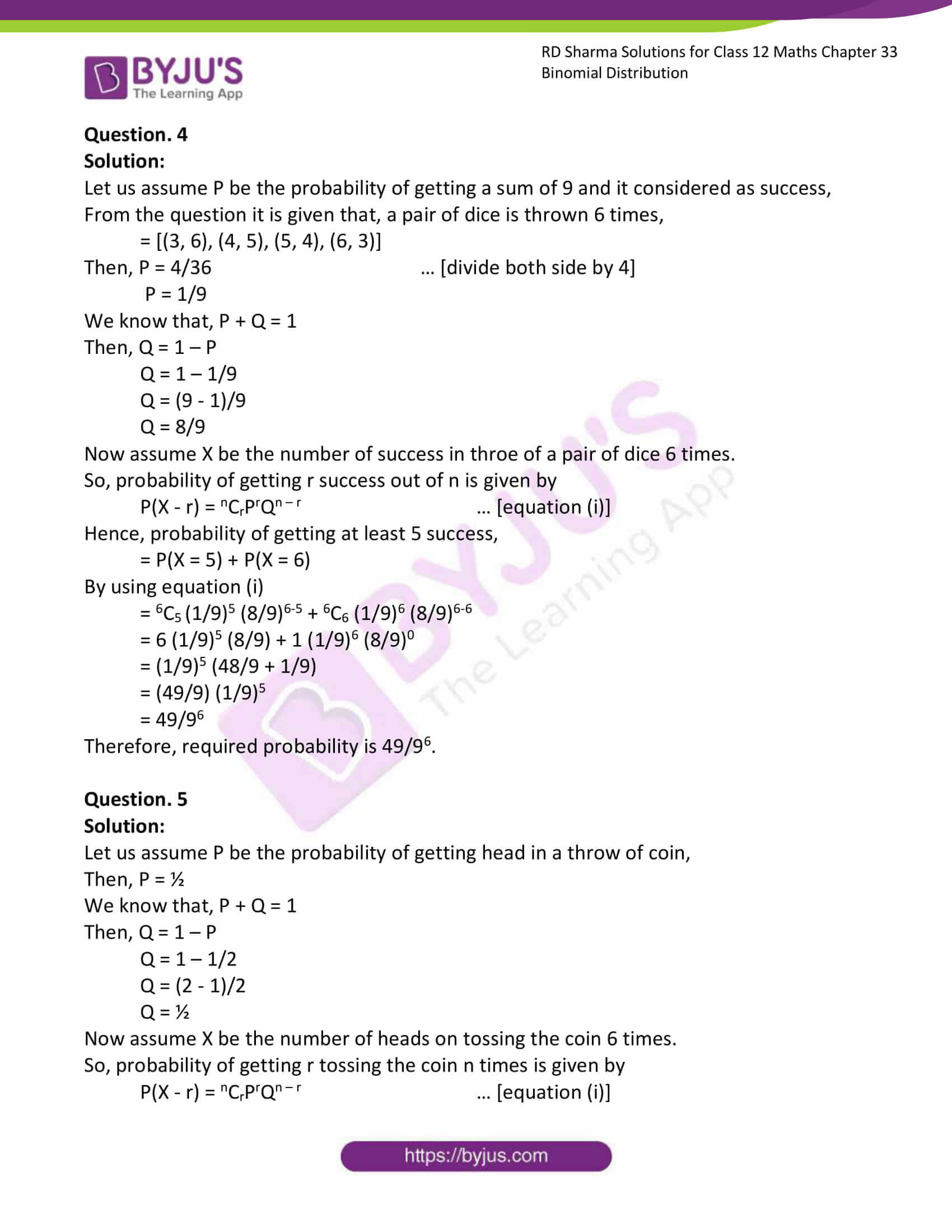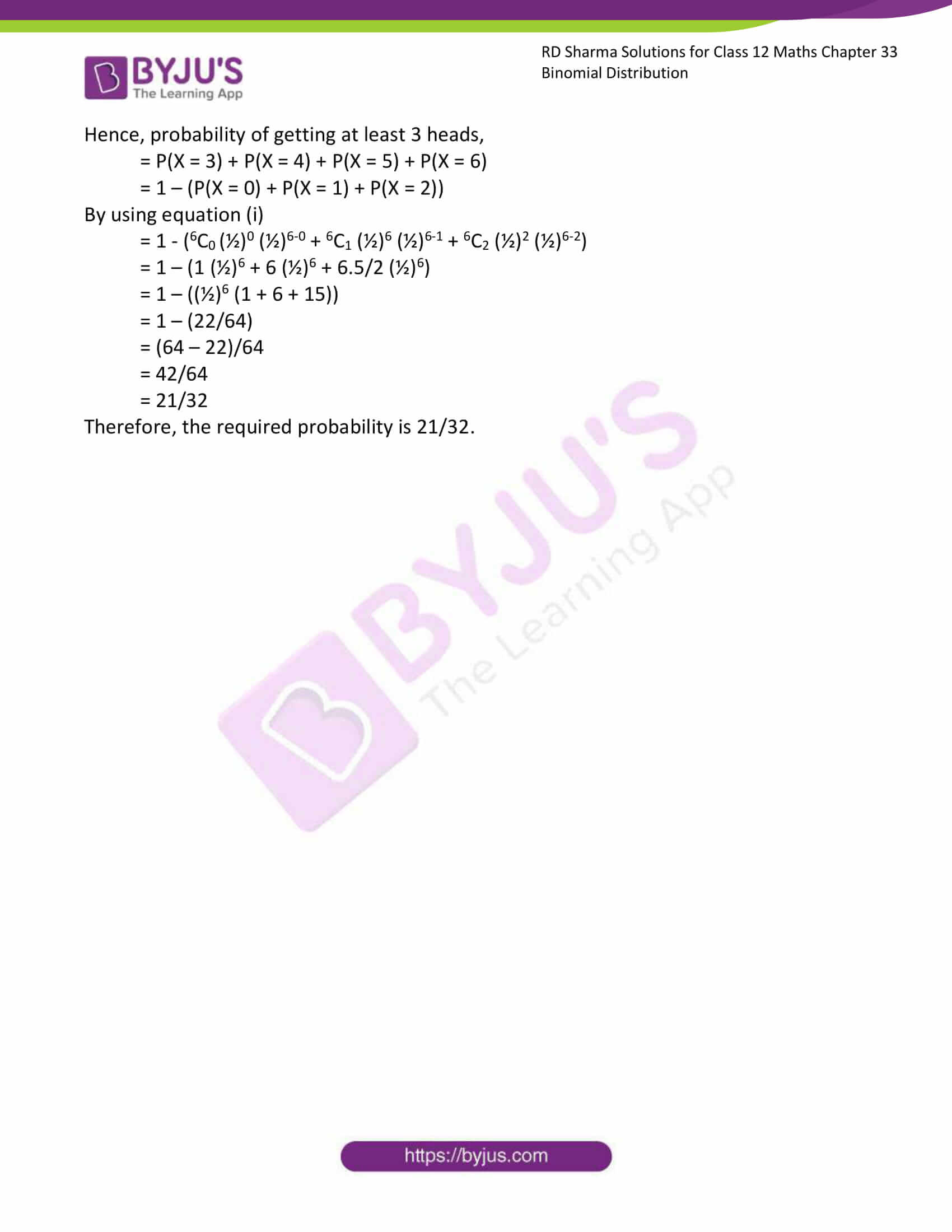# RD Sharma Solutions Class 12 Binomial Distribution Exercise 33.1

RD Sharma Solutions for Class 12 Maths Exercise 33.1 Chapter 33 Binomial Distribution are available here. This exercise of RD Sharma Solutions for Class 12 Maths Chapter 33 contains topics related to Probability. To cover the entire syllabus in Maths, the RD Sharma is an essential material as it offers a wide range of questions that test the students’ understanding of concepts.

## Download PDF of Rd Sharma Solution for Class 12 Maths Chapter 33 Exercise 1### Access Answers for Rd Sharma Solution Class 12 Maths Chapter 33 Exercise 1

EXERCISE 33.1

Question. 1

Solution:

Let us assume P denotes the probability of having defective item,

From the question, P = 6%

= 6/100

P = 3/50

We know that, P + Q = 1

Then, Q = 1 – P

Q = 1 – 3/50

Q = (50 – 3)/50

Q = 47/50

Now assume X denotes the number of defective items in a sample of 8 items.

So, probability of getting r defective bulks is P(X – r) = nCrPrQn – r

P(X – r) = 8Cr(3/50)r (47/50)8 – r … [equation (i)]

Hence, probability of getting not more than one defective item,

= P(X = 0) + P(X = 1)

By using equation (i)

= 8C0(3/50)0 (47/50)8-0 + 8C1 (3/50)1 (47/50)8-1

= 1.1(47/50)8 + 8 (3/50) (47/50)7

= (47/50)7 (47/50 + 24/50)

= (71/50) (47/50)7

= (1.42) (0.94)7

Therefore, the required probability is (1.42) (0.94)7.

Question. 2

Solution:

From the question it is given that,

A coin is tossed 5 times.

So, probability of getting head on one throw of coin = ½

P = ½

We know that, P + Q = 1

Q = 1 – ½

Q = (2 – 1)/2

Q = ½

Now assume X denotes the number of getting head as 5 tosses of coins.

So, probability of getting r heads in n tosses of coin is given by P(X – r) = nCrPrQn – r

P(X – r) = 5Cr(1/2)r (1/2)5 – r … [equation (i)]

Hence, probability of getting at least 3 heads,

= P(X = 3) + P(X = 4) + P(X = 5)

By using equation (i)

= 5C3(½)3 (½)5-3 + 5C4 (½)4 (½)5-4 + 5C5 (½)5 (½)0

= 5C3(½)3 (½)2 + 5C4 (½)4 (½) + 5C5 (½)5 (1)

= (5 × 4)/2 (½)5 + 5 (½)5 + 1 (½)5

= (½)5 (10 + 5 + 1)

= 16 (1/32)

= 16/32

= ½

Therefore, the required probability is ½.

Question. 3

Solution:

Let us assume P denotes the probability of getting tail on a toss of a fair coin,

We know that, P + Q = 1

Then, Q = 1 – P

Q = 1 – ½

Q = (2 – 1)/2

Q = 1/2

Now assume X denotes the number of tail obtained on the toss of coin 5 times.

So, probability of getting r tails in n tosses of coin is given by P(X – r) = nCrPrQn – r

P(X – r) = 5Cr (½)r (½)5 – r … [equation (i)]

Hence, probability of getting tail an odd number of times,

= P(X = 1) + P(X = 3) + P(x = 5)

By using equation (i)

= 5C3(½)1 (½)5-1 + 5C4 (½)3 (½)5-3 + 5C5 (½)5 (½)0

= 5C3(½)1 (½)4 + 5C4 (½)3 (½)2 + 5C5 (½)5 (1)

= (5 × 4)/2 (½)5 + 5 (½)5 + 1 (½)5

= (½)5 (5 + 10 + 1)

= 16 (½)5

= 16(1/32)

= 16/32

= ½

Therefore, the required probability is ½.

Question. 4

Solution:

Let us assume P be the probability of getting a sum of 9 and it considered as success,

From the question it is given that, a pair of dice is thrown 6 times,

= [(3, 6), (4, 5), (5, 4), (6, 3)]

Then, P = 4/36 … [divide both side by 4]

P = 1/9

We know that, P + Q = 1

Then, Q = 1 – P

Q = 1 – 1/9

Q = (9 – 1)/9

Q = 8/9

Now assume X be the number of success in throe of a pair of dice 6 times.

So, probability of getting r success out of n is given by

P(X – r) = nCrPrQn – r … [equation (i)]

Hence, probability of getting at least 5 success,

= P(X = 5) + P(X = 6)

By using equation (i)

= 6C5 (1/9)5 (8/9)6-5 + 6C6 (1/9)6 (8/9)6-6

= 6 (1/9)5 (8/9) + 1 (1/9)6 (8/9)0

= (1/9)5 (48/9 + 1/9)

= (49/9) (1/9)5

= 49/96

Therefore, required probability is 49/96.

Question. 5

Solution:

Let us assume P be the probability of getting head in a throw of coin,

Then, P = ½

We know that, P + Q = 1

Then, Q = 1 – P

Q = 1 – 1/2

Q = (2 – 1)/2

Q = ½

Now assume X be the number of heads on tossing the coin 6 times.

So, probability of getting r tossing the coin n times is given by

P(X – r) = nCrPrQn – r … [equation (i)]

Hence, probability of getting at least 3 heads,

= P(X = 3) + P(X = 4) + P(X = 5) + P(X = 6)

= 1 – (P(X = 0) + P(X = 1) + P(X = 2))

By using equation (i)

= 1 – (6C0 (½)0 (½)6-0 + 6C1 (½)6 (½)6-1 + 6C2 (½)2 (½)6-2)

= 1 – (1 (½)6 + 6 (½)6 + 6.5/2 (½)6)

= 1 – ((½)6 (1 + 6 + 15))

= 1 – (22/64)

= (64 – 22)/64

= 42/64

= 21/32

Therefore, the required probability is 21/32.